## D.3.4 FR2 PAS similarity percentage (PSP)

38.1513GPPNRRelease 17TSUser Equipment (UE) Multiple Input Multiple Output (MIMO) Over-the-Air (OTA) performance requirements

The PSP validation measurements aim at evaluating PAS similarity percentage (PSP), which is one of the validation metrics for characterizing FR2 channel model under test in the quite zone of 3D-MPAC. For PSP validation measurement, only vertical polarization validation is required.

The measurement array is essentially a virtual array configuration realized in 3D-MPAC through a -θ positioning system. The measurement array is a semi-circle and sectored array configuration illustrated in Figure D.3.4-1 where complex channel frequency response is measured at each antenna location 0.5 λ apart using a vector network analyser (VNA) setup. The vertical sectors of the measurement array are limited to 60 (±30) and the horizontal sector to 180 (±90) with the broad side direction points towards the probes. Depending of the turntable architecture/implementation, the virtual array configuration for the PSP validation is composed of two alternative semi-circle arrangements (1 x horizontal and either 2 x crossed vertical or 2 x parallel vertical). The radius of the array element locations with respect to the centre of the test zone is 5 cm, which is equivalent to the half of the test zone radius at 28 GHz. For different frequency bands, the radius of the measurement array sectored semi-circles remains fixed at 5 cm while the spatial sampling of the array varies. This measurement validates the proper angular behaviour in the test zone.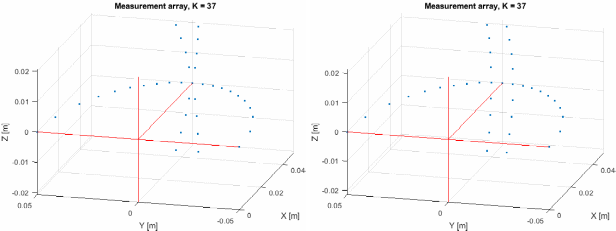Figure D.3.4-1: Semi-circle measurement array configurations with K = 37 elements (at 28 GHz). On the left with two crossed vertical sectors, on the right with two parallel vertical sectors.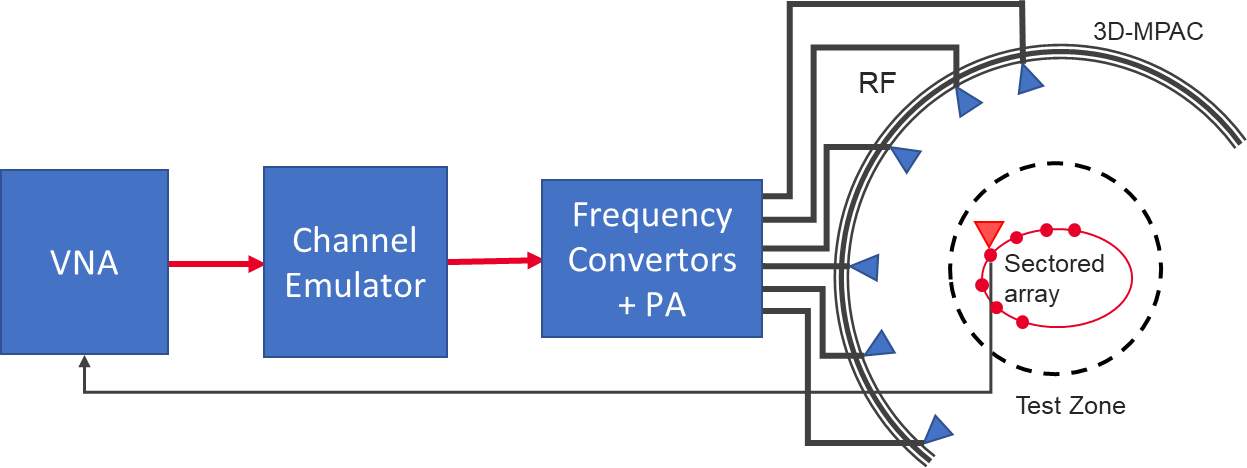Figure D.3.4-2: Setup for FR2 PSP validation measurements

The PSP validation is measured with a vector network analyser as shown in Figure D.3.4-2 illustrating the PSP measurement setup. Port 1 of the VNA transmits signals through the fading emulator and radiate them through L probes within the anechoic chamber. The radiated signals are then received at the test antenna that is positioned inside the test zone. The test antenna is mounted on a -θ positioner which is capable of moving the antenna to pre-defined spatial locations on a fixed radius from the centre of the quiet zone according the measurement array configuration. Finally, the signal is received at port 2 of the VNA. The most suitable approach for the PSP validation is based on an omnidirectional antenna (omnidirectional pattern in AZ and wide BW in EL) as the test can be automated easily. Alternatively, a directional antenna could be used but requires frequent re-positioning.

The measurement and analysis procedure are given as follows:

1. Set the target channel model in the Channel Emulator.

2. For each position of the test antenna on the measurement array configuration in the test zone, step & pause the emulator to different time instances. Measure the complex frequency responses for all stepped channel snapshots , where the interval between frequency and time samples is and, respectively. The number of channel snapshots and frequency samples .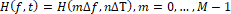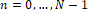3. Move the measurement antenna with a positioner to another location and repeat step 2 to record frequency responses of all stepped channel snapshots.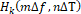4. Repeat step 3 to record frequency responses at all spatial sample points.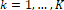5. Estimate the measured PAS through the following two- stage processing:

a. In the first stage, calculate the discrete azimuth and elevation angles (DoA) for the measurement array configuration by applying the MUSIC algorithm. Estimate the powers from the DoA and auto-covariance matrix of the received signal acquired through VNA complex frequency response data.

i) Compose an estimate of the covariance matrix . The entry on the th row and th column of is

where is the complex conjugate operator.

ii) Compose pseudo-PAS of the chamber environment and channel model using the MUSIC algorithm. The intention of this step is to estimate DoAs of signals radiated by probes. Perform first the eigen decomposition of and pick the noise-subspace matrix . The matrix is obtained from the eigen decomposition by removing eigenvectors, i.e. columns, that correspond to X strongest eigenvalues, where X is the number of active probes in the MPAC setup. The pseudo-PAS is 

where is the matrix Hermitean operator and the near-field array factor of the virtual array composed by K spatial measurement antenna locations is

where is the wavelength at the carrier centre frequency, is the norm of a vector, is a location vector of the kth virtual array element, is the unit vector to direction , and R is the a priori known approximate range length . Both vectors and are defined with respect to same origin, which is the centre of the test zone.

iii) Find local maxima of and pick directions , of the X highest peaks.

iv) Perform Bartlett beamforming as defined in step 6 with the steering vector (instead of ) to the X directions identified in iii). The output is X power estimates .

b. In the second stage, use the angle and power estimates, i.e. the discrete PAS of X azimuth and elevation directions and power values in conjunction with a 4×4 DUT sampling array for beamforming with the conventional Bartlett beamformer to estimate the “measured PAS seen by DUT” for PSP calculation. This is

where is the array steering vector of the 4×4 DUT array.

6. Evaluate the reference OTA PAS for the 4×4 DUT array by applying the conventional Bartlett beamformer. The theoretical PAS as reference is calculated for the ideal channel model as

where is the array steering vector of the 4×4 DUT array and is the PAS of the reference channel model.

7. Calculate total variation distance (Dp) from the reference and measured PAS. Mathematically,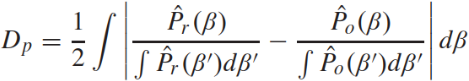8. Calculate PSP values as PSP = (1-Dp) x 100%.

VNA settings:

Table D.3.4-1: VNA settings for FR2 PSP measurements

 Item Unit Value Centre frequency MHz Downlink centre frequencyin Table D.3.1-1 Span MHz 0 (or the minimum) Number of traces 1000 Number of points 1

Channel model specification:

Table D.3.4-2: Channel model specification for FR2 PSP measurements

Item

Unit

Value

Centre frequency

MHz

in Table D.3.1-1

Distance between traces in channel model

wavelength (Note)

> 2

Channel model

As specified in Annex D.1

NOTE: Time [s] = distance [] / MS speed [/s]

MS speed [/s] = MS speed [m/s] / Speed of light [m/s] * Centre frequency [Hz]

Time Domain Alternative Method:

PSP validation can also be implemented using time-domain techniques using the testing setup presented in Figure D.3.4-3. The VNA is substituted by a signal generator, and a signal analyser.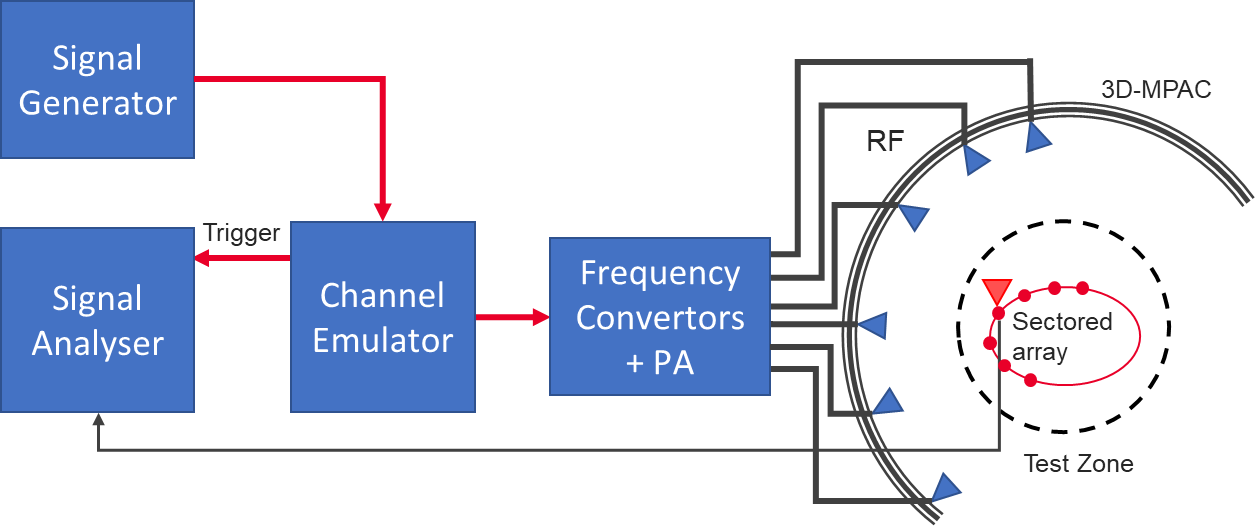Figure D.3.4-3: Setup for FR2 PSP validation measurements based on time domain

Table D.3.4-3: Signal Generator Settings for FR2 PSP measurements based on time domain

 Item Unit Value Centre frequency MHz Downlink centre frequency in Table D.3.1-1 Output power dBm Function of the CE. Sufficiently above Noise Floor

Table D.3.4-4: Signal Analyser Settings for FR2 PSP measurements based on time domain

 Item Unit Value Centre frequency MHz Downlink centre frequency in Table D.3.1-1 Sampling Hz At least 10 times bigger than the max Doppler spread (fd=v/λ) Observation time s At least 32s

The measurement and analysis procedure are given as follows:

Follow the same procedure as before, but M is set to 1. The Channel Emulator is not stepped, but it is allowed to play in free run mode for each of the K spatial points.